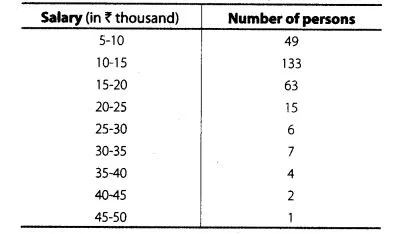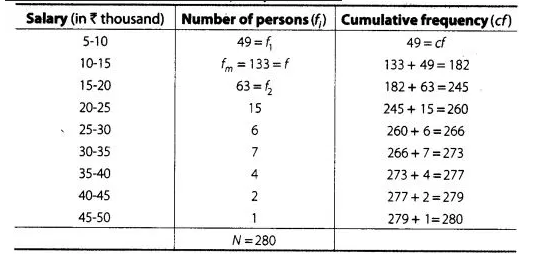# The table below shows the salaries of 280 persons.

Question:

The table below shows the salaries of 280 persons.caluculate the median and mode of the data.

Solution:

First, we construct a cumulative frequency table.$\therefore$ $\frac{N}{2}=\frac{280}{2}=140$

(i) Here, median class is $10-15$, because 140 lies in it.

Lower limit $(l)=10$, Frequency $(f)=133$,

Cumulative frequency $(c f)=49$ and class width $(h)=5$

$\therefore$ Median $=l+\frac{\left(\frac{N}{2}-c f\right)}{f} \times h$

$=10+\frac{(140-49)}{133} \times 5$

$=10+\frac{91 \times 5}{133}$

$=10+\frac{455}{133}=10+3.421$

$=₹ 13.421$ (in thousand)

$=13.421 \times 1000$

$=₹ 13421$

(ii) Here, the highest frequency is 133, which lies in the interval $10-15$, called modal class.

Lower limit $(l)=10$, class width $(h)=5, f_{m}=133, f_{1}=49$, and $f_{2}=63$.

$\therefore$ Mode $=l+\left(\frac{f_{m}-f_{1}}{2 f_{m}-t_{1}-f_{2}}\right) \times h$

$=10+\left\{\frac{133-49}{2 \times 133-49-63}\right\} \times 5$

$=10+\frac{84 \times 5}{266-112}=10+\frac{84 \times 5}{154}=10+2.727$

$=₹ 12.727$ (in thousand)

$=12.727 \times 1000=? 12727$

Hence,the median and modal salary are ₹13421 and ₹12727,respetively.